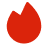# Snapchat人工智能面试真题

BQ

ML Design/ML Case（0）
ML Coding/Data Coding（3）
ML Algorithm（0）
BQ（0）高频题（0）

ML Design/ML Case（0）
ML Coding/Data Coding（3）
ML Algorithm（0）
BQ（0）高频题（0）
1.找长度为K的文件
2.最相似图片
3.最相似朋友
4.最长递增序列
5.LeetCode 289
1. 找长度为K的文件

For exampel.
(0,4,5)-(start point, end point,cost)
(0,6,8)
(2,6.6) -(start is 2,end is 6,and cost is 6)
……
(16,30,6)
(18.30.3)

2. 最相似图片
Suppose you have a set of images, try to find the similar images with a target image. You can define the metrics by yourself
3. 最相似朋友
Given a seed set which is a set of users (100k - 1M) and user profiles (user features) of all the users (500M). output the list of ‘similar' users to the seed set, output should be around 30Mfrom 500M users
4. 最长递增序列
part 1: longest increasing consecutive subsequence
part 2: longest increasing subsequence, need to output the list of the subsequence elements, O(n^2) time complexity,0(1) extra space complexity
5. LeetCode 289
According to Wikipedia's article: "The Game of Life, also known simply as Life, is a cellular automaton devised by the British mathematician John Horton Conway in 1970."

The board is made up of an m x n grid of cells, where each cell has an initial state: live (represented by a 1) or dead (represented by a 0). Each cell interacts with its eight neighbors (horizontal, vertical, diagonal) using the following four rules (taken from the above Wikipedia article):

1. Any live cell with fewer than two live neighbors dies as if caused by under-population.
2. Any live cell with two or three live neighbors lives on to the next generation.
3. Any live cell with more than three live neighbors dies, as if by over-population.
4. Any dead cell with exactly three live neighbors becomes a live cell, as if by reproduction.
The next state is created by applying the above rules simultaneously to every cell in the current state, where births and deaths occur simultaneously. Given the current state of the m x n grid board, return the next state.

Example 1:

```Input: board = [[0,1,0],[0,0,1],[1,1,1],[0,0,0]]
Output: [[0,0,0],[1,0,1],[0,1,1],[0,1,0]]
```
Example 2:

```Input: board = [[1,1],[1,0]]
Output: [[1,1],[1,1]]
```

Constraints:

• m == board.length
• n == board[i].length
• 1 <= m, n <= 25
• board[i][j] is 0 or 1.